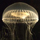## General Question# Brain Teaser - How can you determine if date intervals intersect?

Asked by LostInParadise (31292) June 30th, 2009

Given starting and ending dates for 50 different events, you want to find out if there is any time when all events are happening simultaneously. It can be done using under 100 comparisons. Do you see how?

For those of you who know how to do database queries, assuming a separate record for each event, can you solve the problem with a single select statement?

Observing members: 0Composing members: 0(That would be no.)

Grisaille (12048)“Great Answer” (0) Flag as…I see I have no takers. The answer is not that difficult to come by.

For there to be some time t in every interval means that for each interval, the beginning time b and the end time e must be such that b < t < e. Since all the b values are less than t and all the e values must be greater than t, it follows that all the b values must be less than all the e values. This is also a sufficient condition. If all the b’s are less than all the e’s then the shared time interval is the one between the maximum b and the minimum e. That is how the database query can be framed, comparing the max(beginning time) to the min(end time).

It takes 49 comparisons to find the max b value, another 49 to find the min e value and one additional comparison to compare the two, for a maximum of 99 comparisons. After finding the maximum begin time, instead of finding the maximum end time, simply compare each end time to the the max begin time. In the worst case, it will take 99 comparisons, but if any of the end times come before the max begin time then you can stop comparing and conclude that there is no common interval.

LostInParadise (31292)“Great Answer” (0) Flag as…or